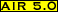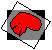# reslice

## Purpose:

This program takes a .air file and uses the information that it contains to load the corresponding image file and generate a new, realigned file.

## Usage:

reslice .air-file output [options]

options:
[-a alternate-reslice-file]
changes the file to be resliced
[-d divide-by-intensity-scale-factor]scales output by dividing by specified factor
[-df divide-by-intensity-scale-factor-file]scales output by dividing by factor specified in file
[-k]
retains the standard file's voxel dimensions (default is to interpolate to cubic voxels)
[-n model {half-window-width(s)}]
changes interpolation model
[-o]
grants permission to overwrite output
[-s multiplicative-intensity-scale-factor]
scales output using specified multiplicative factor
[-sf multiplicative-intensity-scale-factor-file]scales output using multiplicative factor specified in file
[-x dimension size [shift]]
alters output x-dimension, x-voxel size or causes shift of output along the x-axis
[-y dimension size [shift]]
alters output y-dimension, y-voxel size or causes shift of output along the y-axis
[-z dimension size [shift]]
alters output z-dimension, z-voxel size or causes shift of output along the z-axis
where the following definitions apply:
.air-file [mandatory]
name of the input .air file containing the reslice parameters
alternate-reslice-file [-a]
name of an alternate reslice file that is spatially equivalent (same voxel sizes and dimensions) to the default reslice file specified in the .air-file
dimension [-x ...] [-y ...] [-z ...]
the new desired dimension (in voxels)
divide-by-intensity-scale-factor [-d]
output intensities are divided by this positive value
divide-by-intensity-scale-factor-file [-df]
output intensities are divided by the value in this ASCII file containing a single positive number
half-window-width [-n...]
half the width of the window (in whole voxels) to apply when doing sinc interpolation
larger values are more accurate, but considerably slower
model [-n...]
the interpolation model number, selected from the menu:
• 0. nearest neighbor
• 1. trilinear
• 2. windowed sinc in original xy plane, linear along z
• 3. windowed sinc in original xz plane. linear along y
• 4. windowed sinc in original yz plane, linear along x
• 5. 3D windowed sinc
• 6. 3D windowed scanline sinc
• 7. 3D unwindowed scanline sinc
• 10. 3D scanline chirp-z
• 11. scanline chirp-z in original xy plane, linear along z
• 12. scanline chirp-z in original xz plane, linear along y• 13. scanline chirp-z in original yz plane, linear along xmultiplicative-intensity-scale-factor [-s]
output intensities are multiplied by this positive value
multiplicative-intensity-scale-factor-file [-sf]
output intensities are multiplied by the value in this ASCII file containing a single positive number
output [mandatory]
name of the output image file (.hdr or .img suffix optional)
shift [-x ...] [-y ...] [-z ...]
the shift (in voxels) to be applied along this dimension, with positive and negative values causing shifts in opposite directions
size [-x ...] [-y ...] [-z ...]
the new desired voxel size along this dimension (in whatever unit was used for sizes in your image files, usually millimeters)

## Examples:

reslice mri.air newmri
• If files newmri.hdr and newmri.img do not already exist, this command will reslice the reslice file identified in mri.air using the parameters in mri.air. The resulting file will be interpolated to cubic voxels (even if the standard file in mri.air did not have cubic voxels).
reslice mri.air newmri -o
• same as above example but any existing files newmri.hdr and newmri.img will be overwritten
reslice mri.air newmri -o -k
• same as above example except that the output file voxels will have the same dimensions as the standard file (i.e. results will not be cubic voxels unless the standard file itself had cubic voxels)
reslice mri.air newmri -o -k -a mri2
• same as above example except that the file mri2.img will be loaded and resliced instead of the reslice file in mri.air (assuming that mri2 has the same dimensions and sizes as the original reslice file in mri.air)
reslice mri.air newmri -o -z 43 2.25
• The reslice file identified in mri.air will be resliced to generate 43 planes of output data with an interplane distance of 2.25, regardless of the standard file z dimension or voxel z_size.

• The default interpolation model is trilinear interpolation
• Interpolation models 6 and higher all use scanline decomposition to accelerate interpolation. To minimize aliasing, scanline decomposition is preceeded by oversampling of the data in a prepass interpolation step. The AIR package is distributed using a conservative prepass interpolation that doubles the number of voxels along the necessary dimensions, a strategy that should be valid even with fairly large rotations (assuming cubic voxels and a rigid-body spatial transformation model). Prepass interpolation also increases memory requirements and resampling time. As a rough guide, prepass interpolation should be by a factor of (1/cos2(theta)) where theta is the largest expected rigid-body rotation angle (assuming cubic voxels).The issue of aliasing in scanline interpolation is complex and has not been fully addressed for 3D. For a discussion in 2D, see: Fraser, D, Schowengerdt RA. Avoidance of additional aliasing in multipass image rotations. IEEE Transactions on Image Processing 1994;3:721-735.
• If you are using the -z option to specify the number of planes and interplane distance, you may have computed these values to match your scanner's field of view. If you unexpectedly find that data for the last plane is always missing, this may be due to round-off errors. As an exception to the rule otherwise applied in the AIR package when rounding-off voxel sizes (the general rule is round the smallest voxel size downward and all others upwards), here you may need to round the interplane distance downwards to avoid this problem.
• The half window widths for sinc interpolation control the number of surrounding voxels that contribute to the interpolated value along each axis of the reslice file. A half-window width of 1 results in interpolation along that axis that only includes the nearest neighbor on either side and a half-window width of 6 will include the six nearest neighboring voxels in either direction. The total number of voxel included for standard 3D sinc interpolation is therefore 8*(x_half_window_width)*(y_half_window_width*(z_half_window_width). The larger the half windows,the more closely the interpolation will match true sinc interpolation, but also the slower the resampling process. For affine transformations (i.e., for all linear transformations except perspective transformations) the chirp-z interpolation models are generally better choices.
• Windowing of sinc interpolation is implemented using a Hanning window function exactly as described by Hajnal JV, Saeed N, Soar EJ, Oatridge A, Young IR, Bydder GM. Journal of Computer Assisted Tomography 1995:19:289-296.

## Error messages: (alphabetical by case)

-a must be followed by an image file name
• supply an image file name; this file must have the same dimensions and voxel sizes as the reslice file specified in the .air file. The programs scanair and scanheader will allow you to verify this.
-d must be followed by a positive number
• supply a positive number
-df must be followed by a file name
• supply a file name; this must be a plain text ASCII file containing a single number
-k flag cannot be used with the -x, -y or -z flag
• these flags are incompatible; choose one or the other
-n ____ does not define a valid interpolation model
• choose a model number from the menu
-n ____ must be followed by three positive integers
• certain sinc interpolation models require three half-window-widths
-n ____ must be followed by two positive integers
• certain sinc interpolation models require two half-window-widths
-n must be followed by a valid interpolation model number
• choose a model number from the menu
-s must be followed by a positive number
• supply a positive number
-sf must be followed by a file name
• supply a file name; this must be plain text ASCII file containing a single number
-x must be followed by a positive integer and a positive number
• provide the required values, corresponding to the output x-dimension and voxel x-size
-y must be followed by a positive integer and a positive number
• provide the required values, corresponding to the output y-dimension and voxel y-size
-z must be followed by a positive integer and a positive number
• provide the required values, corresponding to the output z-dimensions and voxel z-size
Unable to parse ____
• check syntax for undefined flags
Unable to parse argument ____, which was expected to begin with a '-'
• check syntax, an argument without a flag is positioned as if were a flagModified: December 15, 2001